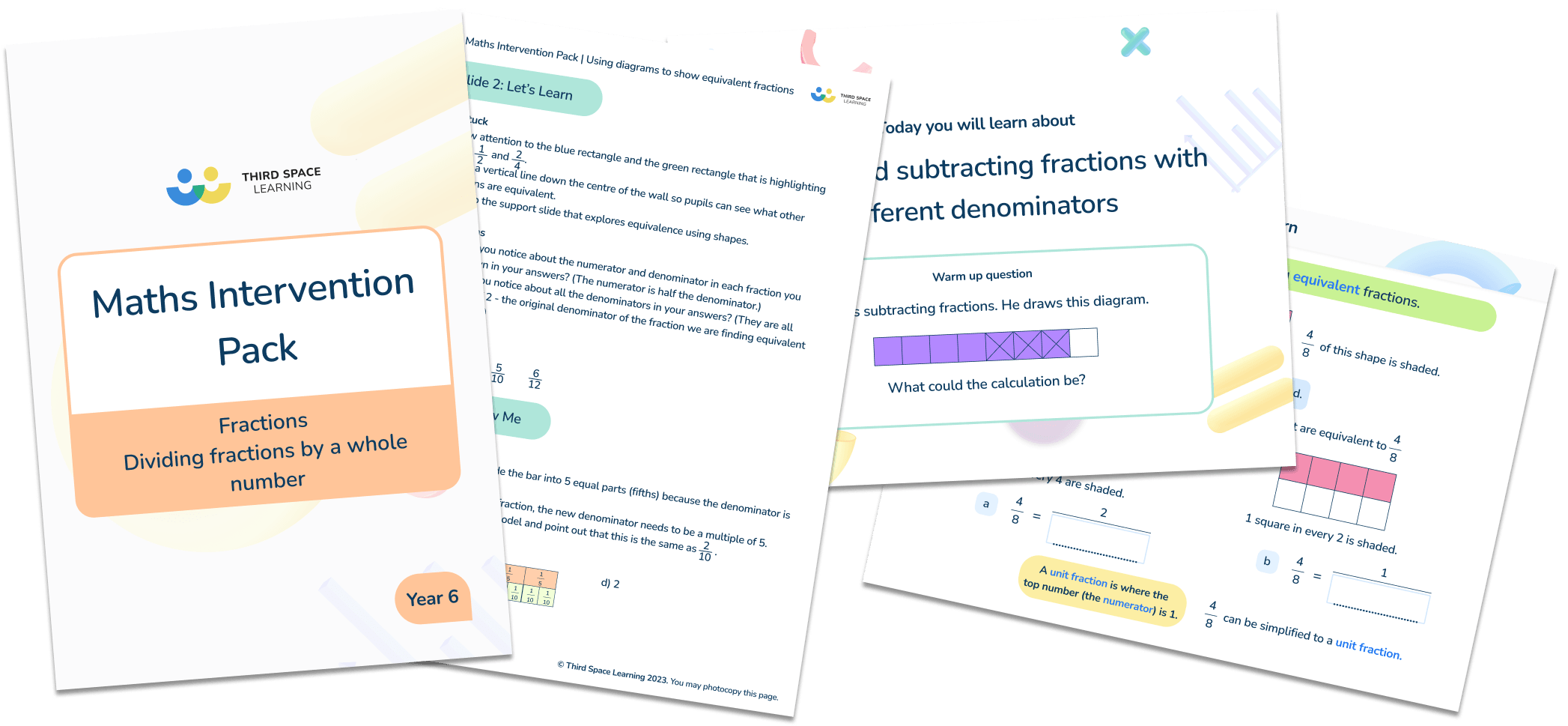# Fractions Intervention Pack

### Years 3 to 6• Lessons include:
• Knowing two quarters is equal to half (Year 2)
• Solving simple problems involving fractions (Year 3)
• Using diagrams to show equivalent fractions (Year 3)
• Finding fractions of an amount (Year 4)
• Simplifying fractions (Year 4)
• Adding and subtracting fractions (Year 5)
• Writing percentages as fractions and decimals (Year 5)
• Dividing fractions by a whole number (Year 6)
• Finding percentages of amounts (Year 6)
• Each lesson contains teaching notes, answers and slides to work through with your pupils.
• Lessons follow exactly the same format that the Third Space Learning specialist maths tutors use in their one to one online maths tutoring.